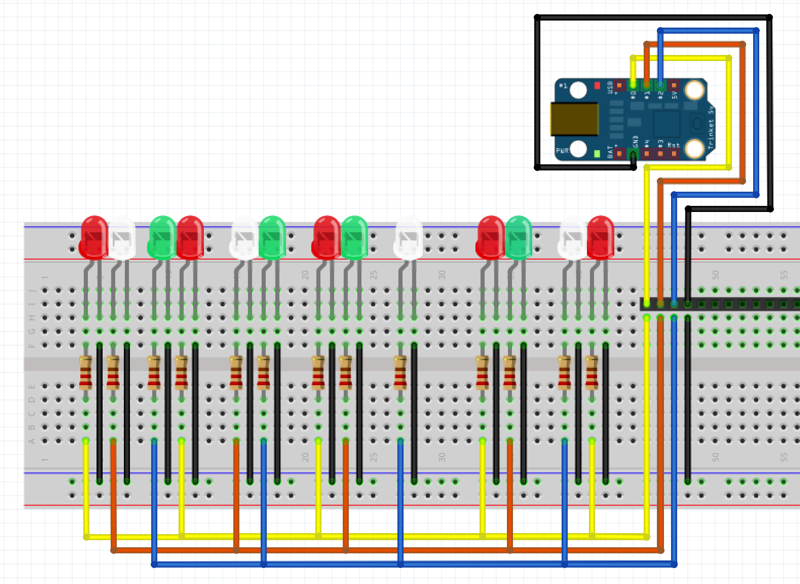## How to calculate resistor values for this project? Moderators: adafruit_support_bill, adafruit

How to calculate resistor values for this project?

Hello,

I am trying to figure out how to calculate the resistor value for the SMD LEDs. There are 3 groups of LEDs I want to control with 3 of the pins on the Trinket (5V).

The LED specs from the seller:
1206 SMD SMT LED
Max. Reverse Current: 10uA
Max. Forward Current: 20Ma
Max. Reverse Voltage: 5V
Forward Voltage: 3.0-3.2VCan somebody help?

If I calculated this correctly, I think I need 100 ohms for each LED.

Thanks.ericbrian

Posts: 22
Joined: Mon Jun 18, 2012 8:52 am

Re: How to calculate resistor values for this project?

Yes. 100 ohms would give you 20mA through the led.Posts: 76150
Joined: Sat Feb 07, 2009 10:11 am

Re: How to calculate resistor values for this project?

I think the max a Trinket pin can supply is 20mA into a load. As drawn, each LED would draw 20mA and so you would have 100mA on each trinket pin which will damage the trinket or cause it to not work. You will need a separate supply and drive each set of 4 leds by using a transistor capable of supporting the 100mA load. Turn the transistor on/off with the Trinket pins.phild13

Posts: 247
Joined: Mon Sep 10, 2012 1:05 pm